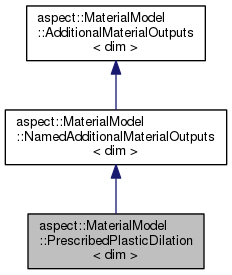ASPECT
aspect::MaterialModel::PrescribedPlasticDilation< dim > Class Template Reference
Inheritance diagram for aspect::MaterialModel::PrescribedPlasticDilation< dim >:[legend]

## Public Member Functions

PrescribedPlasticDilation (const unsigned int n_points)

std::vector< double > get_nth_output (const unsigned int idx) const overridePublic Member Functions inherited from aspect::MaterialModel::NamedAdditionalMaterialOutputs< dim >
NamedAdditionalMaterialOutputs (const std::vector< std::string > &output_names)

NamedAdditionalMaterialOutputs (const std::vector< std::string > &output_names, const unsigned int n_points)

const std::vector< std::string > & get_names () const

void average (const MaterialAveraging::AveragingOperation, const FullMatrix< double > &, const FullMatrix< double > &) overridePublic Member Functions inherited from aspect::MaterialModel::AdditionalMaterialOutputs< dim >

## Public Attributes

std::vector< double > dilationPublic Attributes inherited from aspect::MaterialModel::NamedAdditionalMaterialOutputs< dim >
std::vector< std::vector< double > > output_values

## Detailed Description

### template<int dim> class aspect::MaterialModel::PrescribedPlasticDilation< dim >

An AdditionalOutput that allows prescribing a dilation applied to the Stokes solution.

This is typically used in a MaterialModel to add dilation when plastic failure occurs as motivated by ChoiPeterson2015. If this output (denoted by R below) is present and enable_prescribed_dilation==true the following terms will be assembled:

1) $$\int - (R,q)$$ to the conservation of mass equation, creating $$-(div u,q) = -(R,q)$$. 2) $$\int - 2.0 / 3.0 * eta * (R, div v)$$ to the RHS of the momentum equation (if the model is incompressible), otherwise this term is already present on the left side.

Definition at line 1121 of file interface.h.

## § PrescribedPlasticDilation()

template<int dim>
 aspect::MaterialModel::PrescribedPlasticDilation< dim >::PrescribedPlasticDilation ( const unsigned int n_points )
explicit

Constructor

## § get_nth_output()

template<int dim>
 std::vector aspect::MaterialModel::PrescribedPlasticDilation< dim >::get_nth_output ( const unsigned int idx ) const
overridevirtual

## § dilation

template<int dim>
 std::vector aspect::MaterialModel::PrescribedPlasticDilation< dim >::dilation

A scalar value per evaluation point that specifies the prescribed dilation in that point.

Definition at line 1138 of file interface.h.

The documentation for this class was generated from the following file:
• /home/bob/source/include/aspect/material_model/interface.h